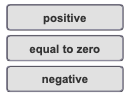# Problem: What is the change in internal energy for the system?Express the energy in kilojoules to two significant figures.Heat (q) is ___ when the system gains thermal energy and ____ when the system loses thermal energy.When work (w) is done by the system, the value is _____ . When work is done on the system, the value is ______ .

###### FREE Expert Solution

Heat (q) is positive when the system gains thermal energy and negative when the system loses thermal energy.

100% (376 ratings)###### Problem Details

What is the change in internal energy for the system?

Express the energy in kilojoules to two significant figures.

Heat (q) is ___ when the system gains thermal energy and ____ when the system loses thermal energy.

When work (w) is done by the system, the value is _____ . When work is done on the system, the value is ______ .